# Compress Machine Fault Recognition Neural Network Using Projection

In this example, you compress a pretrained acoustics-based machine fault recognition neural network using projection and principal component analysis. Then, you generate C++ code from the compressed neural network.

To learn how the deep learning model was trained, see Acoustics-Based Machine Fault Recognition.

For a detailed example on deploying the machine fault recognition system to a hardware target, refer to Acoustics-Based Machine Fault Recognition Code Generation on Raspberry Pi.

### Prerequisites

• MATLAB Coder Interface for Deep Learning Libraries

For supported versions of libraries and for information about setting up environment variables, see Prerequisites for Deep Learning with MATLAB Coder (MATLAB Coder).

### Data Preparation

Download and unzip the air compressor data set . This data set consists of recordings from air compressors in a healthy state or one of seven faulty states.

```downloadFolder = matlab.internal.examples.downloadSupportFile("audio","AirCompressorDataset/AirCompressorDataset.zip"); dataFolder = tempdir; unzip(downloadFolder,dataFolder) dataset = fullfile(dataFolder,"AirCompressorDataset");```

### Create Training and Validation Datastores

Create an `audioDatastore` object to manage the data and split it into training and validation sets.

`ads = audioDatastore(dataset,IncludeSubfolders=true);`

The data labels are encoded in their containing folder name. To split the data into train and test sets, use `folders2labels` and `splitlabels`.

```lbls = folders2labels(ads.Files); idxs = splitlabels(lbls,0.9); adsTrain = subset(ads,idxs{1}); labelsTrain = lbls(idxs{1}); adsValidation = subset(ads,idxs{2}); labelsValidation = lbls(idxs{2});```

Call `countlabels` to inspect the distribution of labels in the train and validation sets.

`countlabels(labelsTrain)`
```ans=8×3 table Label Count Percent _________ _____ _______ Bearing 203 12.5 Flywheel 203 12.5 Healthy 203 12.5 LIV 203 12.5 LOV 203 12.5 NRV 203 12.5 Piston 203 12.5 Riderbelt 203 12.5 ```
`countlabels(labelsValidation)`
```ans=8×3 table Label Count Percent _________ _____ _______ Bearing 22 12.5 Flywheel 22 12.5 Healthy 22 12.5 LIV 22 12.5 LOV 22 12.5 NRV 22 12.5 Piston 22 12.5 Riderbelt 22 12.5 ```

### Extract Training and Validation Features

Extract a set of acoustic features used as inputs to the network. The extraction process is identical to the approach in Acoustics-Based Machine Fault Recognition.

```windowLength = 512; overlapLength = 0; [~,info] = read(adsTrain); fs = info.SampleRate; aFE = audioFeatureExtractor(SampleRate=fs, ... Window=hamming(windowLength,"periodic"),... OverlapLength=overlapLength,... spectralCentroid=true, ... spectralCrest=true, ... spectralDecrease=true, ... spectralEntropy=true, ... spectralFlatness=true, ... spectralFlux=false, ... spectralKurtosis=true, ... spectralRolloffPoint=true, ... spectralSkewness=true, ... spectralSlope=true, ... spectralSpread=true);```

Extract training features.

```reset(adsTrain) trainFeatures = cell(1,numel(adsTrain.Files)); for index = 1:numel(adsTrain.Files) data = read(adsTrain); trainFeatures{index} = (extract(aFE,data))'; end```

Similarly, extract validation features.

```validationFeatures = cell(1,numel(adsValidation.Files)); for index = 1:numel(adsValidation.Files) data = read(adsValidation); validationFeatures{index} = (extract(aFE,data))'; end```

Load the pretrained network. To learn how the deep learning model was trained, see Acoustics-Based Machine Fault Recognition.

`load airCompressorNet`

Display the network layers.

`netOriginal.Layers`
```ans = 6×1 Layer array with layers: 1 'sequenceinput' Sequence Input Sequence input with 10 dimensions 2 'lstm_1' LSTM LSTM with 100 hidden units 3 'dropout' Dropout 20% dropout 4 'lstm_2' LSTM LSTM with 100 hidden units 5 'fc' Fully Connected 8 fully connected layer 6 'softmax' Softmax softmax ```

### Analyze Neuron Activations for Compression Using Projection

You use principal component analysis (PCA) to identify the subspace of learnable parameters that result in the highest variance in neuron activations by analyzing the network activations using the training data set. This analysis requires only the predictors of the training data to compute the network activations. It does not require the training targets.

First, create a `minibatchqueue` object.

• Specify a mini-batch size of 16.

• Specify that the output data has format `"CTB"` (channel, time, batch).

```miniBatchSize = 16; mbqTrain = minibatchqueue(... arrayDatastore(trainFeatures.',OutputType="same",ReadSize=miniBatchSize),... MiniBatchSize=miniBatchSize ,... MiniBatchFormat="CTB",... MiniBatchFcn=@(X)cat(3,X{:}));```

Create a `neuronPCA` object. To view information about the steps of the neuron PCA algorithm, set the `VerbosityLevel` option to` "steps"`.

`npca = neuronPCA(netOriginal,mbqTrain,VerbosityLevel="steps");`
```Using solver mode "direct". Computing covariance matrices for activations connected to: "lstm_1/in","lstm_1/out","lstm_2/in","lstm_2/out","fc/in","fc/out" Computing eigenvalues and eigenvectors for activations connected to: "lstm_1/in","lstm_1/out","lstm_2/in","lstm_2/out","fc/in","fc/out" neuronPCA analyzed 3 layers: "lstm_1","lstm_2","fc" ```

View the properties of the `neuronPCA` object.

`npca`
```npca = neuronPCA with properties: LayerNames: ["lstm_1" "lstm_2" "fc"] ExplainedVarianceRange: [0 1] LearnablesReductionRange: [0 0.9775] InputEigenvalues: {[10×1 double] [100×1 double] [100×1 double]} InputEigenvectors: {[10×10 double] [100×100 double] [100×100 double]} OutputEigenvalues: {[100×1 double] [100×1 double] [8×1 double]} OutputEigenvectors: {[100×100 double] [100×100 double] [8×8 double]} ```

### Project Network

If you want to compress a network so that it meets specific hardware memory requirements, then you can manually calculate the reduction value such that the compressed network is of the desired size.

Specify a target memory requirement of 256 kilobytes (256×1024 bytes).

`targetMemorySize = 256*1024`
```targetMemorySize = 262144 ```

Calculate the memory size of the original network (in bytes) using the `parameterMemory` helper function.

`memorySizeOriginal = parameterMemory(netOriginal)`
```memorySizeOriginal = 502432 ```

Calculate the factor to reduce the learnable parameters by such that the resulting network meets the memory requirements.

`reductionGoal = 1 - (targetMemorySize/memorySizeOriginal);`

Project the network using the `compressNetworkUsingProjection` function and set the `LearnablesReductionGoal` option to the calculated reduction factor.

```netProjected = compressNetworkUsingProjection(netOriginal,npca, ... LearnablesReductionGoal=reductionGoal);```
```Compressed network has 48.0% fewer learnable parameters. Projection compressed 3 layers: "lstm_1","lstm_2","fc" ```

Calculate the memory size of the projected network using the `parameterMemory` function. Notice that the value is very close to the target memory size.

`memorySizeProjected = parameterMemory(netProjected)`
```memorySizeProjected = 261328 ```

### Test Projected Network

Get the expected validation set labels.

```validationLabels = folders2labels(adsValidation.Files); classNames = unique(validationLabels);```

Create a mini-batch queue using the same steps as the training data.

```mbqValidation = minibatchqueue(... arrayDatastore(validationFeatures.',OutputType="same",ReadSize=miniBatchSize),... MiniBatchSize=miniBatchSize ,... MiniBatchFormat="CTB",... MiniBatchFcn=@(X)cat(3,X{:}));```

For comparison, calculate the classification accuracy of the original network using the test data and the `modelPredictions` function.

```YTest = modelPredictions(netOriginal,mbqValidation,string(classNames)); TTest = validationLabels; accOriginal = mean(YTest == TTest)```
```accOriginal = 0.8807 ```

View the confusion chart for the validation data.

```figure confusionchart(YTest,TTest, ... Title="Accuracy: " + accOriginal*100 + " (%)");```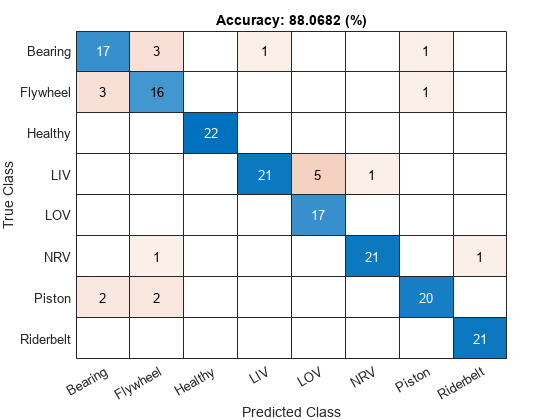Calculate the classification accuracy of the projected network using the test data and the `modelPredictions` function.

```YTest = modelPredictions(netProjected,mbqValidation,string(classNames)); accProjected = mean(YTest == TTest)```
```accProjected = 0.8409 ```

View the confusion matrix of the projected network.

```figure confusionchart(YTest,TTest, ... Title="Accuracy: " + accProjected*100 + " (%)");```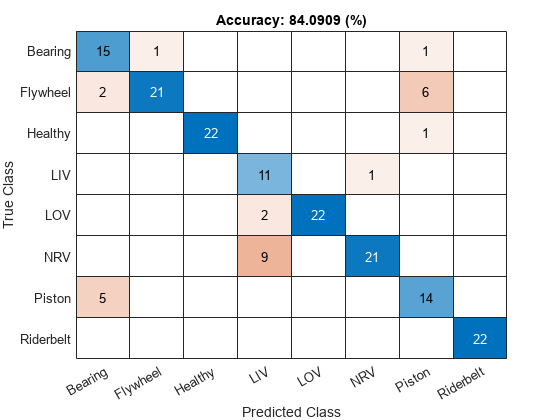Compare the accuracy and the memory size of each network in a bar chart. Notice that memory size has been significantly reduced, with a relatively slight reduction in accuracy.

```figure tiledlayout("flow") nexttile bar([accOriginal accProjected]) xticklabels(["Original" "Projected"]) ylabel("Accuracy (%)") title("Accuracy") nexttile bar([memorySizeOriginal memorySizeProjected]) xticklabels(["Original" "Projected"]) yline(targetMemorySize,"r--","Memory Requirement") ylabel("Memory (bytes)") title("Memory Size")```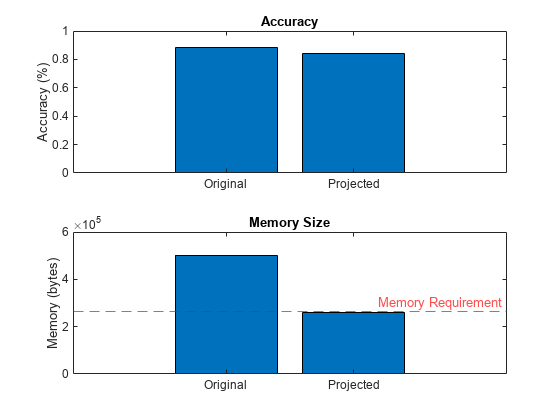### Fine Tune Projected Network

Compressing a network using projection typically reduces the network accuracy. You can improve the accuracy by retraining the network (also known as fine tuning the network).

To define hyperparameters for the network, use `trainingOptions`.

```miniBatchSize =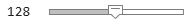128; trainLabels = folders2labels(adsTrain.Files); validationFrequency = floor(numel(trainFeatures)/miniBatchSize); options = trainingOptions("adam", ... MiniBatchSize=miniBatchSize, ... MaxEpochs=35, ... Plots="training-progress", ... Verbose=false, ... Shuffle="every-epoch", ... LearnRateSchedule="piecewise", ... LearnRateDropPeriod=30, ... LearnRateDropFactor=0.1, ... ValidationData={validationFeatures,validationLabels}, ... ValidationFrequency=validationFrequency,... InputDataFormats = "CTB")```
```options = TrainingOptionsADAM with properties: GradientDecayFactor: 0.9000 SquaredGradientDecayFactor: 0.9990 Epsilon: 1.0000e-08 InitialLearnRate: 1.0000e-03 MaxEpochs: 35 LearnRateSchedule: 'piecewise' LearnRateDropFactor: 0.1000 LearnRateDropPeriod: 30 MiniBatchSize: 128 Shuffle: 'every-epoch' WorkerLoad: [] CheckpointFrequency: 1 CheckpointFrequencyUnit: 'epoch' SequenceLength: 'longest' DispatchInBackground: 0 L2Regularization: 1.0000e-04 GradientThresholdMethod: 'l2norm' GradientThreshold: Inf Verbose: 0 VerboseFrequency: 50 ValidationData: {{1×176 cell} [176×1 categorical]} ValidationFrequency: 12 ValidationPatience: Inf CheckpointPath: '' ExecutionEnvironment: 'auto' OutputFcn: [] Metrics: [] Plots: 'training-progress' SequencePaddingValue: 0 SequencePaddingDirection: 'right' InputDataFormats: {'CTB'} TargetDataFormats: "auto" ResetInputNormalization: 1 BatchNormalizationStatistics: 'auto' OutputNetwork: 'last-iteration' ```

To train the network, use `trainnet`.

`fineTunedNet = trainnet(trainFeatures,trainLabels,netProjected,"crossentropy",options);`

### Test Fine-Tuned Network

Calculate the classification accuracy of the fine-tuned network.

```YTest = modelPredictions(fineTunedNet,mbqValidation,string(classNames)); accProjected = mean(YTest == TTest)```
```accProjected = 0.8864 ```

View the confusion matrix of the fine-tuned network.

```figure confusionchart(YTest,TTest, ... Title="Accuracy: " + accProjected*100 + " (%)");```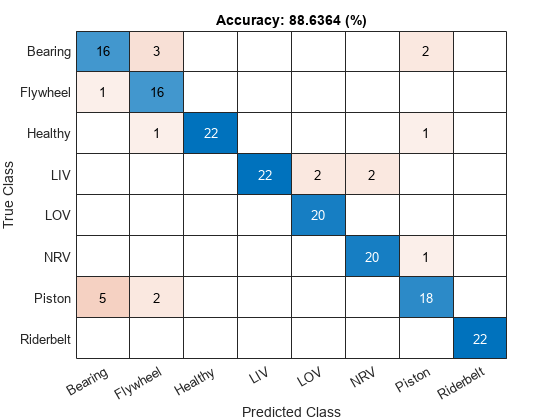### Generate C++ Code from Fine-Tuned Network

You can generate C++ code from a machine fault recognition system that leverages the fine-tuned, compressed network.

The fault recognition system is comprised of two parts:

• Feature extraction.

• Network inference.

#### Create MATLAB Function Compatible with C/C++ Code Generation

Use the `generateMATLABFunction` method of `audioFeatureExtractor` to generate a MATLAB function compatible with C/C++ code generation. Specify `IsStreaming` as `true` so that the generated function is optimized for stream processing.

```filename = fullfile(pwd,"extractAudioFeatures"); generateMATLABFunction(aFE,filename,IsStreaming=true);```

#### Combine Streaming Feature Extraction and Classification

When you compress a network using the `compressNetworkUsingProjection` function, the software replaces layers that support projection with `ProjectedLayer` objects that contain the equivalent neural network. To replace `ProjectedLayer` objects with the corresponding neural networks, use the `unpackProjectedLayers` function.

`fineTunedNet = unpackProjectedLayers(fineTunedNet);`

Save the fine-tuned network to a MAT file.

`save fineTunedNet.mat fineTunedNet`

Create a function that combines the feature extraction and deep learning classification.

`type detectAirCompressorFault.m`
```function scores = detectAirCompressorFault(audioIn) % DETECTAIRCOMPRESSORFAULT This is a streaming classifier function persistent airCompNet if isempty(airCompNet) airCompNet = coder.loadDeepLearningNetwork('fineTunedNet.mat'); end % Extract features using function features = extractAudioFeatures(audioIn); % Classify scores = predict(airCompNet,dlarray(features,'CTB')); end ```

#### Generate C++ Code

Create a code generation configuration object to generate an executable program. Specify the target language as C++.

```cfg = coder.config("mex"); cfg.TargetLang = "C++";```

Create a configuration object for deep learning code generation.

```dlcfg = coder.DeepLearningConfig(TargetLibrary="none"); cfg.DeepLearningConfig = dlcfg;```

Call the `codegen` (MATLAB Coder) function from MATLAB Coder to generate C++ code. Set the input audio frame length to 512 samples.

```audioFrame = ones(512,1,"single"); codegen -config cfg detectAirCompressorFault -args {audioFrame} -report```
```Code generation successful: View report ```

For a more detailed example on deploying the machine fault recognition system to a hardware target, refer to Acoustics-Based Machine Fault Recognition Code Generation on Raspberry Pi.

### Supporting Functions

```function Y = modelPredictions(net,mbq,classNames) Y = []; reset(mbq) while hasdata(mbq) X = next(mbq); scores = predict(net,X); labels = onehotdecode(scores,classNames,1)'; Y = [Y; labels];%#ok end end function N = numLearnables(net) N = 0; for i = 1:size(net.Learnables,1) N = N + numel(net.Learnables.Value{i}); end end function numBytes = parameterMemory(net) numBytes = 4*numLearnables(net); end```

References

 Verma, Nishchal K., et al. "Intelligent Condition Based Monitoring Using Acoustic Signals for Air Compressors." IEEE Transactions on Reliability, vol. 65, no. 1, Mar. 2016, pp. 291–309. DOI.org (Crossref), doi:10.1109/TR.2015.2459684.